# Swift Tutorial Part 1: Expressions, Variables and Constants

Welcome to our mini-series on getting started with programming in Swift! In this series, you’ll learn some Swift programming basics using playgrounds.

#### Version

• Swift 4.2, iOS 12, Xcode 10
Update note: Lorenzo Boaro updated this tutorial to iOS 12, Xcode 10, and Swift 4.2. Matt Galloway wrote the original.

Welcome to our mini-series on getting started with programming in Swift!

In this series, you’ll learn some of the basics of programming while working in a modern, friendly environment with the Swift programming language.

Instead of us boring you with a lot of theory, you’ll get to coding right away by using Swift Playgrounds, which are sandbox-type environments wherein you can run code directly without having to code an entire app.

For this tutorial, you’ll need Xcode 10, the standard development environment for macOS, available here. If you have Xcode version 9 or below, some of the code in this tutorial won’t work as expected.

## Getting Started

The set of tools that you use to write software is often referred to as the tool chain. The part of the tool chain into which you write your code is known as the Integrated Development Environment (IDE). Xcode is your IDE, which includes playgrounds.

You’ll use playgrounds throughout this series to practice coding, so it’s important to understand how they work. That’s what you’ll learn during the rest of this tutorial.

## Creating a Playground

When you open Xcode, it will greet you with the following welcome screen: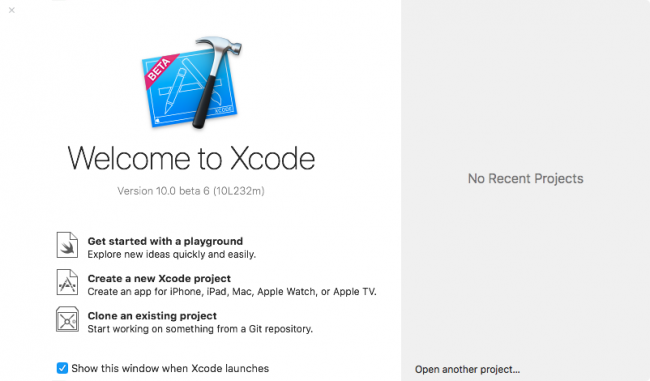If you don’t see this screen, it’s most likely because the Show this window when Xcode launches option was unchecked. You can also open the screen by pressing Command-Shift-1. You can also click WindowWelcome to Xcode from the menu bar.

From the Welcome screen, you can jump quickly into a playground by clicking on Get started with a playground. Click on that now and Xcode will present you with a choice of templates.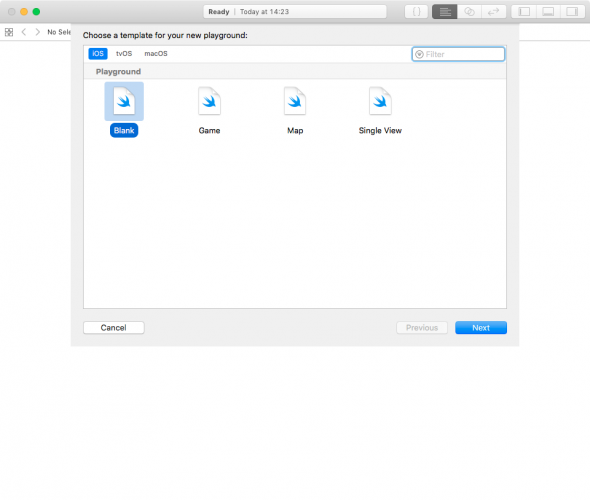The platform you choose simply defines which version of the template Xcode will use to create the playground. Currently, your options are iOS, macOS or tvOS. Each platform comes with its own environment set up and ready for you to begin playing around inside with code. For the purposes of this tutorial, choose whichever platform you wish. You won’t be write any platform-specific code; instead, you’ll learn the core principles of the Swift language.

Select the Blank template and click Next. Xcode will now ask you to name the playground and select a location to save it. The name is merely cosmetic and for your own use; when you create your playgrounds, feel free to choose names that will help you remember what they’re about.

Click Create to create and save the playground. Xcode then presents you with the playground, like so: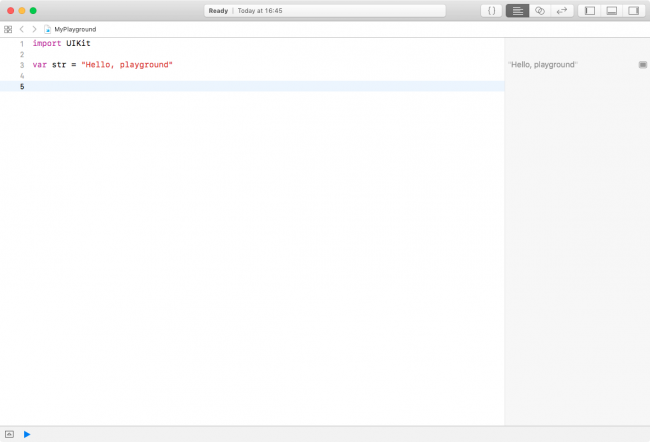New playgrounds don’t start entirely empty but have some basic starter code to get you going. Don’t worry — you’ll soon learn what this code means.

### Playgrounds Overview

At first glance, a playground may look like a rather fancy text editor. Well, here’s some news for you: It’s essentially just that!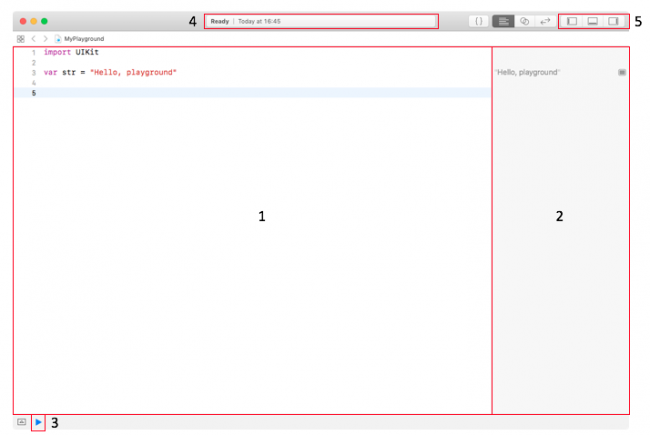The screenshot above highlights the first and most important things to know about the following:

1. Source editor: This is the area in which you’ll write your Swift code. It’s much like a text editor such as Notepad or TextEdit. You’ll notice that it uses what’s known as a monospace font, which means all characters are the same width. This makes the code much easier to read and format.
2. Results sidebar: This area shows the results when you execute your code. You’ll learn more about how code is executed as you read through the series. The results sidebar is the main place that you’ll look to confirm your code is working as expected.
3. Execution control: Playgrounds execute automatically by default, meaning you can write code and immediately see the output. This control allows you to execute the playground again. Holding down the ▶︎ (Execute) button allows you to switch between automatic execution and manual execution modes.
4. Activity viewer: This shows the status of the playground. In the screenshot above, it shows that the playground has finished executing and is ready to handle more code in the source editor. When the playground is executing, the viewer will indicate this with a spinner.
5. Panel controls: These toggle switches show and hide three panels, one that appears on the left, one on the bottom and one on the right. The panels each display extra information that you may need to access from time to time. You’ll usually keep them hidden, as they are in the screenshot. You’ll learn more about each of these panels as you move through the series.

Playgrounds execute the code in the source editor from top to bottom. Every time you change the code, the playground will re-execute everything. You can also force a re-execution by clicking EditorRun Playground in the menu bar. Alternatively, you can use the execution control.

You can turn on line numbers on the left side of the source editor by clicking XcodePreferences…Text EditingLine Numbers in the menu bar. Line numbers can be very useful when you want to refer to parts of your code.

Once the playground finishes execution, Xcode updates the results sidebar to show the results of the corresponding lines in the source editor. You’ll see how to interpret the results of your code as you work through the examples in this series.

Code comments, arithmetic operations, constants and variables are some of the fundamental building blocks of any language, and Swift is no different.

The Swift compiler generates executable code from your source code. To accomplish this, it uses a detailed set of rules. Sometimes these details can obscure the bigger picture of why you wrote your code a certain way or even what problem you are solving. To prevent this, it’s good to document what you wrote so that the next human who passes by will be able to make sense of your work. That next human, after all, may be future you! :]

Swift, like most other programming languages, allows you to document your code through the use of what are called comments. These allow you to write any text directly alongside your code, which is ignored by the compiler.

The first way to write a comment is like so:

```// This is a comment. It is not executed.
```

This is a single line comment. You could stack these up like so to allow you to write paragraphs:

```// This is also a comment.
// Over multiple lines.
```

However, there is a better way to write comments that span multiple lines. Like so:

```/* This is also a comment.
Over many...
many...
many lines. */
```

This is a multi-line comment. The start is denoted by `/*` and the end is denoted by `*/`. Simple!

You should use code comments where necessary to document your code, explain your reasoning or simply to leave jokes for your colleagues. :]

## Printing Out

It’s also useful to see the results of what your code is doing. In Swift, you can achieve this through the use of the `print` command.

`print` will output whatever you want to the debug area, which is sometimes referred to as the console.

For example, consider the following code:

```print("Hello, Swift Apprentice reader!")
```

This will output a nice message to the debug area, like so: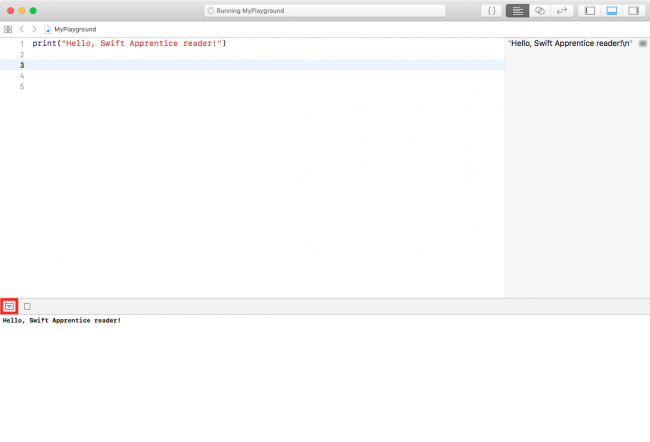You can hide or show the debug area using the downward-arrow-in-a-box button highlighted in red in the image above. You can also click ViewDebug AreaShow Debug Area in the menu bar to do the same thing.

## Arithmetic Operations

When you take one or more pieces of data and turn them into another piece of data, this is known as an operation.

The simplest way to understand operations is to think about arithmetic. The addition operation takes two numbers and converts them into the sum of the two numbers. The subtraction operation takes two numbers and converts them into the difference of the two numbers.

You’ll find simple arithmetic all over your apps: From tallying the number of “likes” on a post to calculating the correct size and position of a button or a window, numbers are indeed everywhere!

In this section, you’ll learn about the various arithmetic operations that Swift has to offer by considering how they apply to numbers. Later, you’ll see operations for types other than numbers.

### Simple Operations

All operations in Swift use a symbol known as the operator to denote the type of operation they perform.

Consider the four arithmetic operations that you learned in your early school days: addition, subtraction, multiplication and division. For these simple operations, Swift uses the following operators:

• Add: `+`
• Subtract: `-`
• Multiply: `*`
• Divide: `/`

These operators are used like so:

```2 + 6

10 - 2

2 * 4

24 / 3
```

Each of these lines is what is known as an expression. An expression has a value. In these cases, all four expressions have the same value: 8. You write the code to perform these arithmetic operations much as you would write it if you were using pen and paper.In your playground, you can see the values of these expressions in the right-hand bar, known as the results sidebar, like so: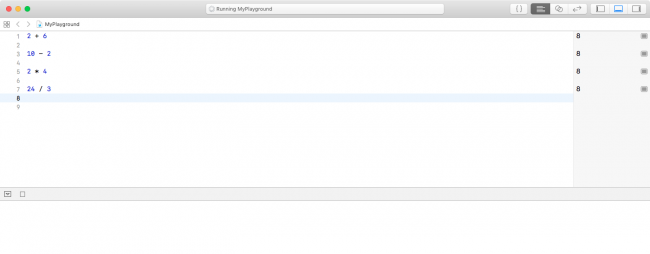If you want, you can remove the whitespace surrounding the operator:

```2+6
```

Removing the whitespace is an all-or-nothing thing: You can’t mix styles. For example:

```2+6   // OK
2 + 6 // OK
2 +6  // ERROR
2+ 6  // ERROR
```

It’s often easier to read expressions if you have whitespace on either side of the operator.

### Decimal Numbers

All of the operations above have used whole numbers, more formally known as integers. However, as you will know, not every number is whole.

As an example, consider the following:

```22 / 7
```

This, you may be surprised to know, results in the number 3. This is because, if you only use integers in your expression, Swift makes the result an integer. In this case, the result is rounded down to the next integer.

You can tell Swift to use decimal numbers by changing it to the following:

```22.0 / 7.0
```

This time, the result is 3.142857142857143, as expected.

### The Remainder Operation

The four operations you’ve seen so far are easy to understand because you’ve been doing them for most of your life. Swift also has more complex operations you can use, all of them standard mathematical operations — just less common ones. You’ll review them, now.

The first of these is the remainder operation, also called the modulo operation. In division, the denominator goes into the numerator a whole number of times, plus a remainder. This remainder is exactly what the remainder operation gives. For example, 10 modulo 3 equals 1, because 3 goes into 10 three times, with a remainder of 1.

In Swift, the remainder operator is the `%` symbol, and you use it like so:

```28 % 10
```

In this case, the result equals `8`, because 10 goes into 28 twice with a remainder of 8. If you want to compute the same thing using decimal numbers, you do it like so:

```(28.0).truncatingRemainder(dividingBy: 10.0)
```

This computes divides 28 by 10 and then truncates the result, chopping off any extra decimals, and it returns the remainder of that. The result is identical to % when there are no decimals.

### Shift Operations

The shift-left and shift-right operations take the binary form of a decimal number and shift the digits left or right, respectively. They then return the decimal form of the new binary number.

For example, the decimal number 14 in binary, padded to 8 digits, is `00001110`. Shifting this left by two places results in `00111000`, which is 56 in decimal.

Here’s an illustration of what happens during this shift operation: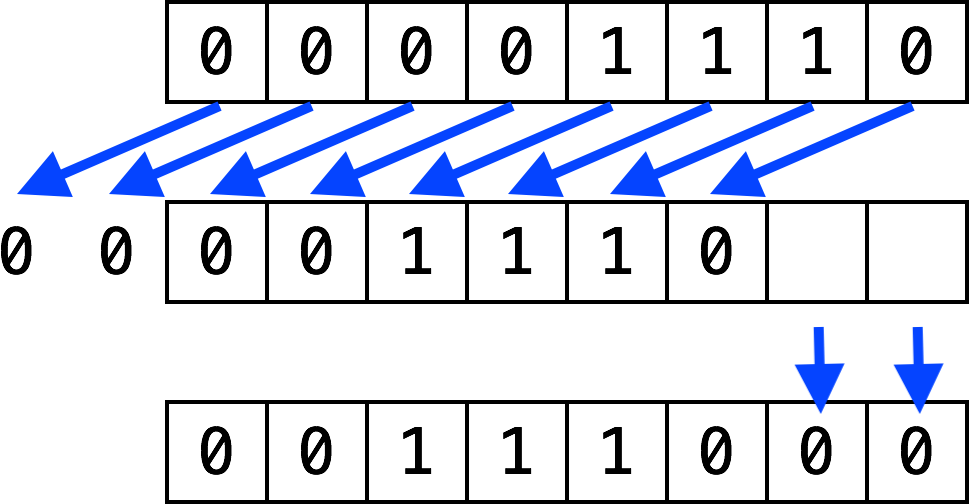The digits that come in to fill the empty spots on the right become 0. The digits that fall off the end on the left are lost.

Shifting right is the same, but the digits move to the right.

The operators for these two operations are as follows:

• Shift left: `<<`
• Shift right: `>>`

These are the first operators you’ve seen that contain more than one character. Operators can contain any number of characters, in fact.

Here’s an example that uses both of these operators:

```1 << 3

32 >> 2
```

Both of these values equal the number 8.

One reason for using shifts is to make multiplying or dividing by powers of two easy. Notice that shifting left by one is the same as multiplying by two, shifting left by two is the same as multiplying by four, and so on. Likewise, shifting right by one is the same as dividing by two, shifting right by two is the same as dividing by four, and so on.

In the old days, code often made use of this trick because shifting bits is much simpler for a CPU to do than complex multiplication and division arithmetic. Therefore, the code was faster if it used shifting. However, these days, CPUs are much faster and compilers can even convert multiplication and division by powers of two into shifts for you. So you’ll see shifting only for binary twiddling, which you probably won’t see unless you become an embedded systems programmer!

### Order of Operations

There are many times that it is necessary to use multiple operators to calculate a value. Here’s an example of how to do this in Swift:

```((8000 / (5 * 10)) - 32) >> (29 % 5)
```

Notice the use of parentheses, which, in Swift, serve two purposes: to make it clear to anyone reading the code — including yourself — what you meant, and to disambiguate. For example, consider the following:

```350 / 5 + 2
```

Does this equal 72 (350 divided by 5, plus 2) or 50 (350 divided by 7)? Those of you who paid attention in school will be screaming “72!” And you would be right!

Swift uses the same reasoning and achieves this through what’s known as operator precedence. The division operator (`/`) has a higher precedence than the addition operator (`+`), so, in this example, the code executes the division operation first.

If you wanted Swift to do the addition first — that is, to return 50 — then you could use parentheses like so:

```350 / (5 + 2)
```

The precedence rules follow the same that you learned in math class at school. Multiply and divide have the same precedence, higher than add and subtract, which also have equal precedence.

## Math Functions

Swift also has a vast range of math functions for you to use when necessary. You never know when you need to pull out some trigonometry, especially when you’re a pro Swifter and writing those complex games!

Note: Not all of these functions are part of Swift. Some are provided by the operating system (OS). Don’t remove the `import` statement that comes as part of the playground template or Xcode will tell you it can’t find these functions.

For example, consider the following:

```sin(45 * Double.pi / 180)
// 0.7071067811865475

cos(135 * Double.pi / 180)
// -0.7071067811865475
```

These compute the sine and cosine, respectively. Notice how both make use of `Double.pi`, which is a constant Swift provides you, ready-made with pi, calculated to as much precision as is possible by the computer. Neat!

Then there’s this:

```(2.0).squareRoot() // Same as sqrt(2)
// 1.414213562373095
```

This computes the square root of 2. Did you know that sin(45°) equals 1 over the square root of 2?

Another common operation is to find the max of two variables. You can use them like so:

```max(5, 10)
// 10

min(-5, -10)
// -10
```

These compute the maximum and minimum of two numbers respectively.

If you’re particularly adventurous, you can even combine these functions like so:

```max(sqrt(2), Double.pi / 2)
// 1.570796326794897
```

## Naming Data

At its simplest, computer programming is all about manipulating data. Remember, everything you see on your screen can be reduced to numbers that you send to the CPU. Sometimes, you yourself represent and work with this data as various types of numbers; other times, the data comes in more complex forms such as text, images and collections.

In your Swift code, you can give each piece of data a name that you use to refer to it later. The name carries with it an associated type that denotes what sort of data the name refers to, such as text, numbers or a date.

You’ll learn about some of the basic types next, and you’ll encounter many other types throughout the rest of this series.

### Constants

Take a look at this:

```let number: Int = 10
```

This declares a constant called `number`, which is of type `Int`. Then, it sets the value of the constant to the number `10`.

Note: Thinking back to operators, here’s another one: The equals sign, `=`, is known as the assignment operator.

The type `Int` can store integers. The way you store decimal numbers is like so:

```let pi: Double = 3.14159
```

This is similar to the `Int` constant, except the name and the type are different. This time, the constant is a `Double`, a type that can store decimals with high precision.

There’s also a type called `Float`, short for floating point, that stores decimals with lower precision than `Double`. In fact, `Double` has about double the precision of `Float`, which is why it’s called `Double` in the first place. A `Float` takes up less memory than a `Double` but, generally, memory use for numbers isn’t a huge issue and you’ll see `Double` used in most places.

Once you’ve declared a constant, you can’t change its data. For example, consider the following code:

```let number: Int = 10
number = 0
```

This code produces an error:

`Cannot assign to value: 'number' is a 'let' constant`

In Xcode, you would see the error represented this way: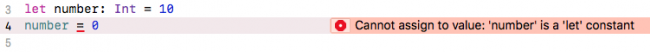Constants are useful for values that aren’t going to change. For example, if you were modeling an airplane and needed to keep track of the total number of seats available, you could use a constant.

You might even use a constant for something like a person’s age. Even though their age will change with each birthday, you might only be concerned with their age at this particular instant.

### Variables

Often, you want to change the data behind a name. For example, if you were keeping track of your bank account balance with deposits and withdrawals, you might use a variable rather than a constant.

If your program’s data never changed, then it would be a rather boring program! But, as you’ve seen, it’s not possible to change the data behind a constant.

When you know you’ll need to change some data, you should use a variable to represent that data instead of a constant. You declare a variable in a similar way, like so:

```var variableNumber: Int = 42
```

Only the first part of the statement is different: You declare constants using `let`, whereas you declare variables using `var`.

Once you’ve declared a variable, you’re free to change it to whatever you wish, as long as the type remains the same. For example, to change the variable declared above, you could do this:

```variableNumber = 0
variableNumber = 1_000_000
```

To change a variable, you simply assign it a new value.

Note: In Swift, you can optionally use underscores to make larger numbers more human-readable. The quantity and placement of the underscores are up to you.

This is a good time to take a closer look at the results sidebar of the playground. When you type the code above into a playground, you’ll see that the results sidebar on the right shows the current value of `variableNumber` at each line: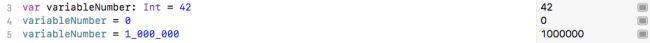The results sidebar will show a relevant result for each line if one exists. In the case of a variable or constant, the result will be the new value, whether you’ve just declared a constant, or if you've declared or reassigned a variable.

### Using Meaningful Names

Always try to choose meaningful names for your variables and constants. Good names, like good comments, can make your code easier to read.

A good name specifically describes what the variable or constant represents. Here are some examples of good names:

• `personAge`
• `numberOfPeople`
• `gradePointAverage`

Often, a bad name is simply not descriptive enough. Here are some examples of bad names:

• `a`
• `temp`
• `average`

The key is to ensure that you’ll understand what the variable or constant refers to when you read it again later. Don’t make the mistake of thinking you have an infallible memory! It’s common in computer programming to look back at your own code as early as a day or two later and have forgotten what it does. Make it easier for yourself by giving your variables and constants intuitive, precise names.

Also, note how the names above are written. In Swift, it is common to camel case names, explained below. For variables and constants, follow these rules to properly case your names:

• If the name is made up of multiple words, join them together and start each subsequent word with an uppercase letter.
• If one of these words is an abbreviation, write the entire abbreviation in the same case (e.g., `sourceURL` and `urlDescription`).

In Swift, you can even use the full range of Unicode characters. For example, you could declare a variable like so:

```var 🐶💩: Int = -1
```

That might make you laugh, but use caution with special characters like these. They are harder to type and, therefore, may end up causing you more pain than amusement.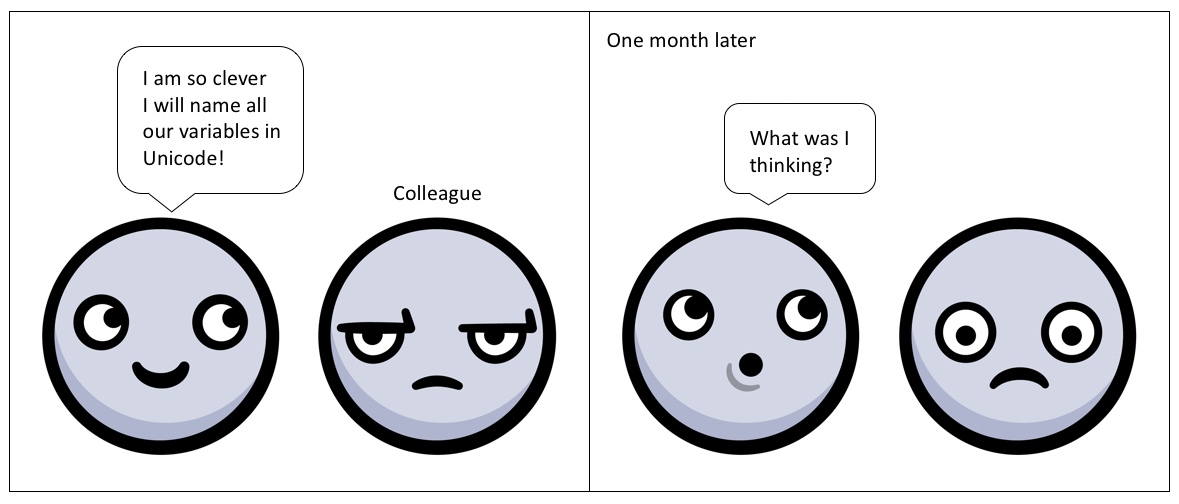Special characters like these probably make more sense in data that you store rather than in Swift code.

## Increment and Decrement

A common operation that you will need is to be able to increment or decrement a variable. In Swift, this is achieved like so:

```var counter: Int = 0

counter += 1
// counter = 1

counter -= 1
// counter = 0
```

The `counter` variable begins as `0`. The increment sets its value to `1`, and then the decrement sets its value back to `0`.

These operators are similar to the assignment operator (`=`), except that they also perform an addition or subtraction. They take the current value of the variable, add or subtract the given value, and assign the result to the variable.

In other words, the code above is shorthand for the following:

```var counter: Int = 0

counter = counter + 1
// counter = 1

counter = counter - 1
// counter = 0
```

Similarly, the `*=` and `/=` operators do the equivalent for multiplication and division, respectively:

```var counter: Int = 10

counter *= 3 // Same as counter = counter * 3
// counter = 30

counter /= 2 // Same as counter = counter / 2
// counter = 15
```

## Where to Go From Here?

You can download the final playground using the Download Materials button at the top or bottom of this tutorial. To improve your Swift skills, you will find some mini-exercises to complete. If you are stuck or you need some help, feel free to take advantage of companion solutions.

In this tutorial, you’ve only dealt with numbers — both integers and decimals. Of course, there’s more to the world of code than that! In the next part of the series, you’re going to learn about more types such as strings, which allow you to store text.

The next part in this tutorial series deals with types and operations; continue on to Part 2: Types and Operations to carry on with your Swift adventures!

If have any questions or comments, please tell us in the discussion below!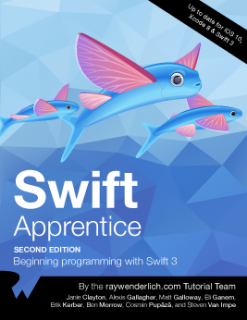This tutorial was taken from Chapters 1 and 2 of Swift Apprentice, Fourth Edition, available from the raywenderlich.com store.

Check it out and let us know what you think!